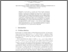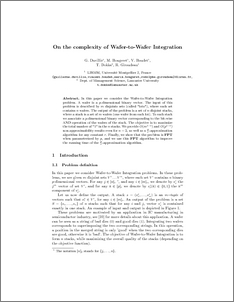# On the complexity of wafer-to-wafer integration

Duvillié, Guillerme and Bougeret, Marin and Boudet, Vincent and Dokka Venkata Satyanaraya, Trivikram and Girodeau, Rodolpe (2015) On the complexity of wafer-to-wafer integration. In: Algorithms and Complexity. Lecture Notes in Computer Science . Springer, Cham, pp. 208-220. ISBN 9783319181721Preview
PDF (CIAC2015)
CIAC2015.pdf - Accepted Version

## Abstract

In this paper we consider the Wafer-to-Wafer Integration problem. A wafer is a p -dimensional binary vector. The input of this problem is described by m disjoints sets (called “lots”), where each set contains n wafers. The output of the problem is a set of n disjoint stacks, where a stack is a set of m wafers (one wafer from each lot). To each stack we associate a p -dimensional binary vector corresponding to the bit-wise AND operation of the wafers of the stack. The objective is to maximize the total number of “1” in the n stacks. We provide O(m 1−ϵ ) and O(p 1−ϵ ) non-approximability results even for n=2 , as well as a pr -approximation algorithm for any constant r . Finally, we show that the problem is FPT when parameterized by p , and we use this FPT algorithm to improve the running time of the pr -approximation algorithm.

Item Type:
Contribution in Book/Report/Proceedings
Departments:
ID Code:
76944
Deposited By:
Deposited On:
01 Dec 2015 09:02
Refereed?:
Yes
Published?:
Published